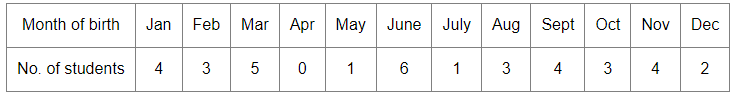# The table given below shows the month of birth of 36 students of a class.

Question:

The table given below shows the month of birth of 36 students of a class.A student is chosen at random from the class. What is the probability that the chosen student was born in October?

(a) $\frac{1}{3}$

(b) $\frac{2}{3}$

(c) $\frac{1}{4}$

(d) $\frac{1}{12}$

Solution:

(d) $\frac{1}{12}$

Explanation:
Total number of students = 36
Number of students born in October = 3

Let E be the event that the chosen student was born in October. Then,

$P(E)=\frac{\text { Number of students born in October }}{\text { Total number of students }}=\frac{3}{36}=\frac{1}{12}$